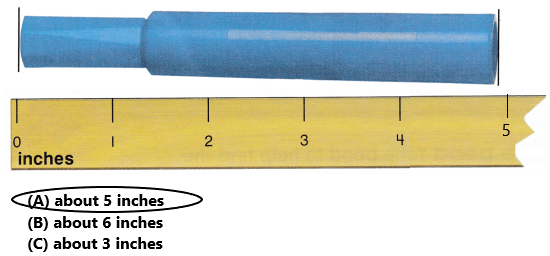# Texas Go Math Grade 2 Lesson 16.2 Answer Key Measure with an Inch Ruler

Refer to our Texas Go Math Grade 2 Answer Key Pdf to score good marks in the exams. Test yourself by practicing the problems from Texas Go Math Grade 2 Lesson 16.2 Answer Key Measure with an Inch Ruler.

## Texas Go Math Grade 2 Lesson 16.2 Answer Key Measure with an Inch Ruler

Explore

The bead is 1 inch long. Use the bead to help find the length of each string. Draw to show what you did.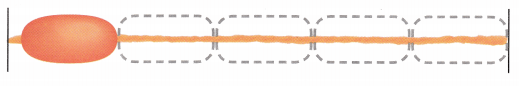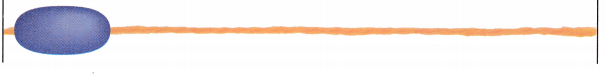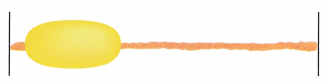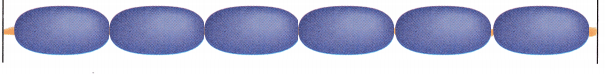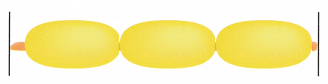Explanation:
The length of 1st string is 5 inches long
The length of the 2nd string is 6 inches long and
The length of the 3rd string is 3 inches long.

FOR THE TEACHER • Have children use the picture to help them predict how many beads will fit on each string. Then, without measuring, ask them to estimate the length of each string.

Math Talk
Mathematical Processes

Describe how the bead helped you find the length of each string.

Model and Draw

What is the length of the string to the nearest inch?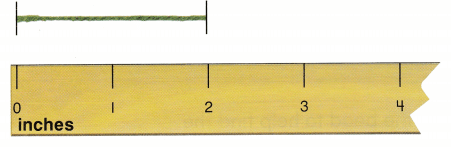STEP 1:
Line up the end of the string with the zero mark on the ruler.

STEP 2:
Find the inch mark that is closest to the other end of the string.
___________ inches
I lined up the end of the string with the zero mark on the ruler
The inch mark that is closest to the other end of the string is 2
Therefore, the string is 2 inches long.

Share and Show

Measure the length to the nearest inch.

Question 1.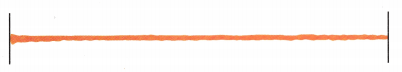___________ inches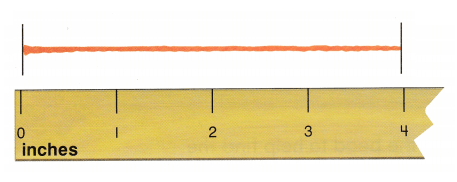Explanation:
I lined up the end of the string with the zero mark on the ruler
The inch mark that is closest to the other end of the string is 4
Therefore, the string is 4 inches long.

Question 2.___________ inchesExplanation:
I lined up the end of the string with the zero mark on the ruler
The inch mark that is closest to the other end of the string is 5
Therefore, the string is 5 inches long.

Question 3.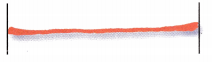___________ inches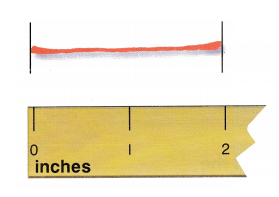Explanation:
I lined up the end of the string with the zero mark on the ruler
The inch mark that is closest to the other end of the string is 2
Therefore, the string is 2 inches long.

Question 4.___________ inches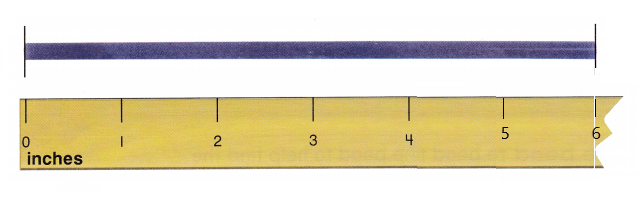Explanation:
I lined up the end of the string with the zero mark on the ruler
The inch mark that is closest to the other end of the string is 6
Therefore, the string is 6 inches long.

Problem Solving

Measure the length to the nearest inch.

Question 5.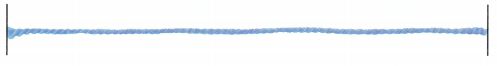___________ inches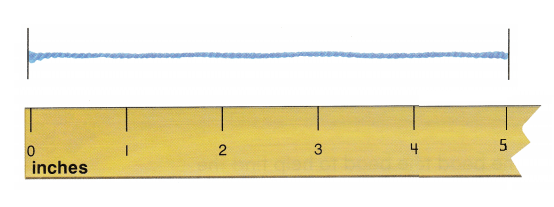Explanation:
I lined up the end of the string with the zero mark on the ruler
The inch mark that is closest to the other end of the string is 5
Therefore, the string is 5 inches long.

Question 6.___________ inches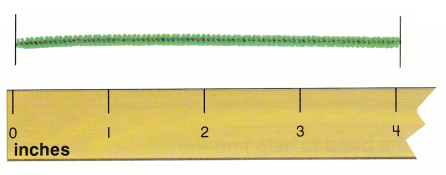Explanation:
I lined up the end of the string with the zero mark on the ruler
The inch mark that is closest to the other end of the string is 4
Therefore, the string is 4 inches long.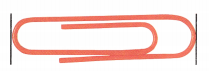___________ inches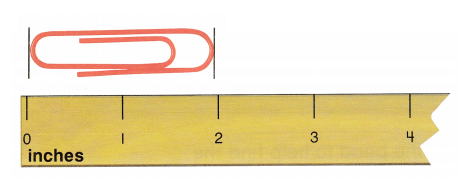Explanation:
I lined up the end of the paper clip with the zero mark on the ruler
The inch mark that is closest to the other end of the paper clip is 2
Therefore, the paper clip is 2 inches long.

Solve.Question 8.
H.O.T. Multi-Step How much longer is the red string than the blue string?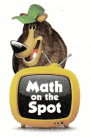_________ inches longer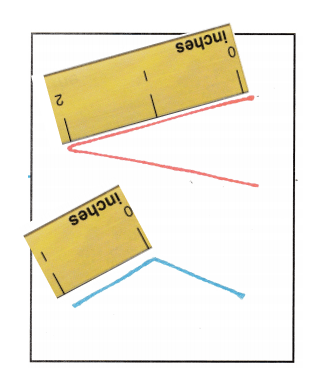Explanation:
The red string is 2+2 = 4 inches long, the blue string is 1+1 = 2 inches long
The red string is 4-2= 2 inches longer than blue string
Therefore, the red string is 2 inches longer than the blue string.

Question 9.
H.O.T. If the red and blue strings were straight and placed end to end, what would the total length be?
_________ inches
6 inches

Explanation:
If the red and blue strings were straight and placed end to end
Add the lengths of both the strings that is 4+2 = 6
Therefore, the total length will be 6 inches long.

Question 10.
Use Tools Use an inch ruler. What is the length of the ribbon to the nearest inch?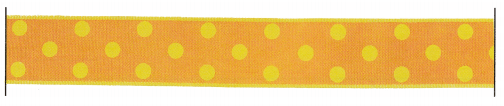(A) 5 inches
(B) 2 inches
(C) 4 inches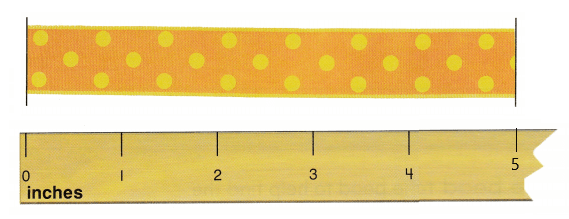Explanation:
I lined up the end of the ribbon with the zero mark on the ruler
The inch mark that is closest to the other end of the ribbon is 5
Therefore, the ribbon is 5 inches long.

Question 11.
Apply Use an inch ruler. What is the length of the paper clip to the nearest inch?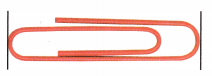(A) 4 inches
(B) 1 inch
(C) 2 inches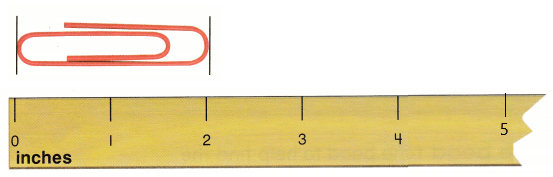Explanation:
I lined up the end of the paper clip with the zero mark on the ruler
The inch mark that is closest to the other end of the paper clip is 2
Therefore, the paper clip is 2 inches long.

Question 12.
Texas Test Prep Use an inch ruler. What is the length of the pencil to the nearest inch?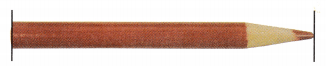(A) 1 inch
(B) 4 inches
(C) 3 inches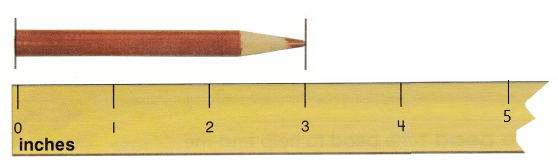Explanation:
I lined up the end of the pencil with the zero mark on the ruler
The inch mark that is closest to the other end of the pencil is 3
Therefore, the pencil is 3 inches long.

TAKE HOME ACTIVITY • Have your child measure the lengths of some objects to the nearest inch using a ruler or a similar measuring tool.

### Texas Go Math Grade 2 Lesson 16.2 Homework and Practice Answer Key

Measure the length to the nearest inch.

Question 1.__________ inchesThe length of the ribbon is about 4 inches long.
Explanation:
I lined up the end of the ribbon with the zero mark on the ruler.
The inch mark that is closest to the other end of the ribbon is 4.
Therefore, the ribbon is about 4 inches long.

Question 2.__________ inchesThe length of the yarn is about 5 inches long.
Explanation:
I lined up the end of the yarn with the zero mark on the ruler.
The inch mark that is closest to the other end of the yarn is 5.
Therefore, the yarn is 5 inches long.

Question 3.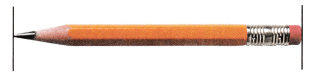__________ inchesThe length of the pencil is about 3 inches long.
Explanation:
I lined up the end of the pencil with the zero mark on the ruler.
The inch mark that is closest to the other end of the pencil is 3.
Therefore, the pencil is 3 inches long.

Problem Solving

Question 4.
Multi-Step Measure the lengths to the nearest inch. How much shorter is the ribbon than the yarn?____________ inch shorterThe ribbon is 1 inch shorter than yarn.
Explanation:
I lined up the end of the yarn and ribbon with the zero mark on the ruler.
The inch mark that is closest to the other end of the yarn is 4.
The inch mark that is closest to the other end of the ribbon is 3.
The yarn is about 4 inches long. The ribbon is about 3 inches long. So, the ribbon is 1 inch shorter than yarn.

Lesson Check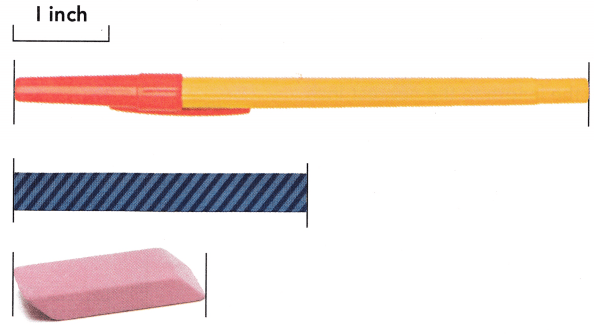Question 5.
Jack chooses an object that is about 2 inches long. Which object did Jack choose?
(A) pen
(B) ribbon
(C) eraser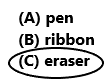Option C is correct.
Explanation:
In the above image we can observe three different objects. Jack chooses an object that is about 2 inches long. Jack chosen eraser. Because in all the three objects eraser is about 2 inches long. So, draw a circle for option C.

Question 6.
Jane chooses an object that is about 3 inches long. Which object did Jane choose?
(A) pen
(B) ribbon
(C) eraserOption B is correct.
Explanation:
In the above image we can observe three different objects. Jack chooses an object that is about 3 inches long. Jack chosen ribbon. Because in all the three objects ribbon is about 3 inches long. So, draw a circle for option B.

Question 7.
Use an inch ruler. What is the length of the marker to the nearest inch?